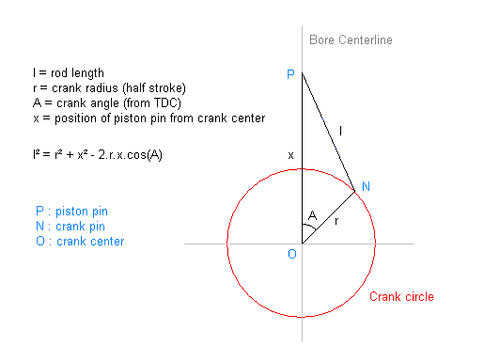# Crank mechanism design

TheRedDevil18
I want to design a crank mechanism. I found this page on Wikipedia
https://en.wikipedia.org/wiki/Piston_motion_equationsRelating from this diagram, is x the max stroke of the piston ?, or is it PO-(max stroke of piston) ?

And why is l^2 = r^2 + x^2 - 2*r*x*cos(A)

Shouldn't it be, l^2 = r^2 + OP^2 - 2*r*OP*cos(A) ?